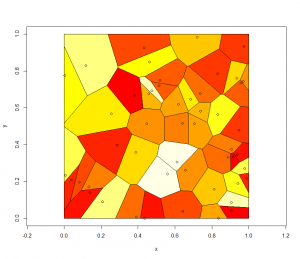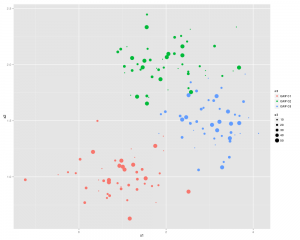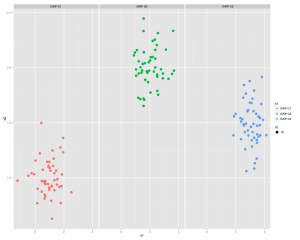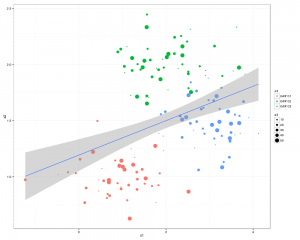# Some Basic DNF

```sudo dnf repolist
sudo dnf config-manager --enablerepo=fedora-multimedia
sudo dnf config-manager --disablerepo=fedora-multimedia
```

That last one will ignore all mono packages from your currently identified upgrade list.

# Moving Files By Name

…where the name is made up of the date and time.

I’ve got a lot of image files that I tend to dump into whatever directory I feel like.
This simple python script will create directories and move files around based on the file name, as long as the filename follows the following format YYYYMM. Bascially as long as the first 6 characters are integers it should work. In my file system that’s fine In yours the same may not be true. If the first 6 characters is not an integer then it will leave them alone.

Notice I’m not checking for JPG/MP4 files only; I’m not checking that the year or month are valid values; I’m not checking the EXIF values and basing it on them if the filename is not valid; there are lots of really cool things I could do as well but I don’t need those because that’s how my files are set out. I’m not building in lots of features that don’t touch my use-case.

```import os
import shutil

path = '/home/mike/TestPython/'
os.chdir(path)

for file in os.walk(path):
for name in file:
year = name[:4]
month = name[4:6]
if name[:6].isdigit():
if not os.path.exists(year):
os.makedirs(year)
if not os.path.exists(year + "/" + month):
os.makedirs(year + "/" + month)
src = file + "/" + name
dst = path + year + "/" + month + "/"+name
shutil.move(src, dst)
```

Once you’ve moved all the files into their new homes delete all the empty directories. This code has been ever so slightly modifed from this StakOverflow post. It was modified for Python 3, by adding brackets to the print syntax:

```import os
currentDir = '/home/mike/TestPython/'

index = 0
for root, dirs, files in os.walk(currentDir):
for dir in dirs:
newDir = os.path.join(root, dir)
index += 1
print (str(index) + " ---> " + newDir)
try:
os.removedirs(newDir)
print("Directory empty! Deleting...")
print(" ")
except:
print("Directory not empty and will not be removed")
print(" ")
```

# Simple outlook inbox analysis using R

Emails from eBay members asking questions come through as randomstring@members.ebay.com which can make them hard to count as a group
The following R script re-codes them so they can be counted.

```install.packages("plyr")
library(plyr)

setwd("/home/mike/Desktop")
dir()
names(inbox)

"members.ebay.co.uk" ,

str(inbox)

plot(f\$N)
str(f)
```

Once you've identified the biggest culprits, make a rule to move or delete them from your email.

# Voronoi in R (from deldir manual)```install.packages("deldir")

install.packages("deldir")
library(deldir)
set.seed(42)
x <- runif(50)
y <- runif(50)
z <- deldir(x,y,rw=c(0,1,0,1))
w <- tile.list(z)
plot(w)
ccc <- heat.colors(20) # Or topo.colors(20), or terrain.colors(20)
# or cm.colors(20), or rainbow(20).
```

# Recoding a factor

When you have N levels of a factor but you would like M (M < N) you need to recode the data set.

when you run str(df) you get an idea that factors are numbered in any vetor or data frame.

We need to use a command to recode the levels. The command you use is ‘levels’:

```levels(df\$factor)[c(2,4,6,7)] = "Horse Whispering"
```

Which means: Take levels that have the internal numberings of 2,4,6,7 and convert them to being “Horse Whispering”.
To recode the rest you need to find the internal numbering of the new levels for the df:

```levels(df\$factor)
```

because the levels that were formally 2,4,6 and 7 have now been recoded into a single value and you’ll have to adjust the integers that you are using every time you run the command.

Continue on until all the necessary coding has been completed.

To make sure you have recoded properly you should make a copy of the first factor and recode the copy rather than the original. That way you can compare new and old later:

```table(df\$OrigFactor , df\$RecodedFactor)
```

Which will print out a table of counts for OrigFactor Vs RecodedFactor

# ggplot2/qplot basics

Install and load the ggplot2 and Cairo libraries

```install.packages(c("ggplot2","Cairo")
library(c(ggplot2,Cairo))
```

set up some data (or use some real data)

```x1<-rnorm(150,mean = rep(1:3, each =50),sd = 0.7)
x2<-rnorm(150,mean = rep(c(1,2,1.5), each = 50),sd = 0.2)
x3<-rnorm(150,mean = rep(c(20,30,3),each = 50)), sd = 0.5)
n3<-rep(c("GRP 01","GRP 02","GRP 03"),each=50)
```

Here is the command to generate the PNG file, with anti-aliasing:

```CairoPNG(filename = "Plot1.png", antialias="subpixel", width = 1000, height=800, units = "px")
{
qplot(x1,x2, ,color = n3, size = x3)
}
dev.off()
```or you can split the 3 sections up using:

` qplot(x1,x2, color = n3, facets = .~n3)`# ...and now something similar using GGPLOT2

First thing we need to do is create a dataframe from the four identical length vectors.

```df <- data.frame(x1,x2,x3,n3)
colnames(df) <- c("x1","x2","x3","n3")```

Some Charting:

```g1 <- ggplot(df,aes(x1,x2))
p <- g1 + geom_point(aes(colour=n3), size =3.5) +
geom_smooth(method = "lm") +
theme_bw()
print(p)
```

..and a slightly better looking version:

```g1 <- ggplot(df,aes(x1,x2))
p  <- g1 + geom_point(aes(colour=n3, size =x3)) +
geom_smooth(method = "lm") +
theme_bw()
print(p)
```There you go all good stuff.
Other things to check out: facet_wrap
Some more pretty graphics

# create a vector of dates in R Cran

```dt <- as.Date('2010-01-01')
dts <- seq(dt,length =17, by="+1 month")
```

# GDELT data into ms-sql

Go…here

Use the following script to create a database in the required location:

```CREATE TABLE GDELT_HISTORICAL (
GLOBALEVENTID bigint , --1
SQLDATE int,
MonthYear char(6) ,
[Year] char(4) ,
FractionDate decimal , --5
Actor1Code char(55) ,
Actor1Name char(255) ,
Actor1CountryCode char(55) ,
Actor1KnownGroupCode char(55) ,
Actor1EthnicCode char(55) , --10
Actor1Religion1Code char(55) ,
Actor1Religion2Code char(55) ,
Actor1Type1Code char(55) ,
Actor1Type2Code char(55) ,
Actor1Type3Code char(55) ,
Actor2Code char(55) , --16
Actor2Name char(255) ,
Actor2CountryCode char(55) ,
Actor2KnownGroupCode char(55) ,
Actor2EthnicCode char(55) ,
Actor2Religion1Code char(55) ,
Actor2Religion2Code char(55) ,
Actor2Type1Code char(55) ,
Actor2Type2Code char(55) ,
Actor2Type3Code char(55) ,
IsRootEvent int ,
EventCode char(4) ,
EventBaseCode char(4) ,
EventRootCode char(4) ,
GoldsteinScale decimal ,
NumMentions int ,
NumSources int ,
NumArticles int ,
AvgTone decimal ,
Actor1Geo_Type int  ,
Actor1Geo_FullName char(255) ,
Actor1Geo_CountryCode char(2) ,
Actor1Geo_Lat float ,
Actor1Geo_Long float ,
Actor1Geo_FeatureID int  ,
Actor2Geo_Type int  ,
Actor2Geo_FullName char(255) ,
Actor2Geo_CountryCode char(2) ,
Actor2Geo_Lat float ,
Actor2Geo_Long float ,
Actor2Geo_FeatureID int  ,
ActionGeo_Type int  ,
ActionGeo_FullName char(255) ,
ActionGeo_CountryCode char(2) ,
ActionGeo_Lat float ,
ActionGeo_Long float ,
ActionGeo_FeatureID float  ,
);
```

Unzip all your history files into one location and then run this script for each file:

```  BULK INSERT GDELT_HISTORICAL
FROM 'C:\Users\MONKEYMIKE\Desktop\201302.csv'
WITH
(
FIELDTERMINATOR = '\t'
, ROWTERMINATOR = '0x0a'--'\n'
)
```

# treemap in R

```library(RODBC)
library(lattice)
library(treemap)

ch<-odbcConnect("mike_db",uid="mike")
c<-sqlQuery(ch, paste("select"
,"ward,year(end_Dttm) as [year]"
,",sum(datediff(mi,start_Dttm,end_Dttm)/1440.0) as LOS"
,"from [wardstays_examples]"
,"GROUP BY ward ,year(end_Dttm)"
))
str(c)

treemap (c
,index=c("year","ward") # the different levels
,vSize = "LOS" # the value on which to scale the squares
)

```